# Weierstrass criterion (for uniform convergence)

A theorem which gives sufficient conditions for the uniform convergence of a series or sequence of functions by comparing them with appropriate series and sequences of numbers; established by K. Weierstrass . If, for the series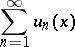of real- or complex-valued functions defined on some setthere exists a convergent series of non-negative numbers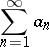such that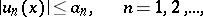then the initial series converges uniformly and absolutely on the set(cf. Absolutely convergent series). For instance, the series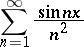converges uniformly and absolutely on the entire real axis, since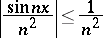and the seriesis convergent.

If, for a sequence of real- or complex-valued functions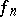,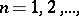which converges to a functionon a setthere exists a sequence of numbers,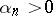andas, such that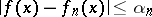,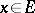,then the sequence converges uniformly on. For instance, the sequence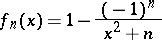converges uniformly to the functionon the entire real axis, since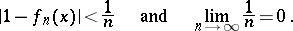The Weierstrass criterion for uniform convergence may also be applied to functions with values in normed linear spaces.